Question

# QUESTION 2 (20 MARKS) (a) A continuous time signal x(t) = 3e2tu(-t) is an input to...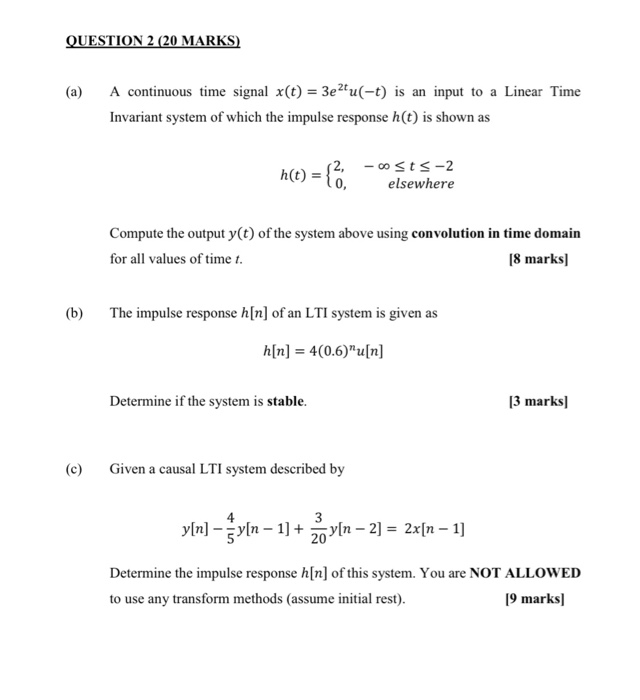QUESTION 2 (20 MARKS) (a) A continuous time signal x(t) = 3e2tu(-t) is an input to a Linear Time Invariant system of which the impulse response h(t) is shown as h(t) = { .. 12, -osts-2 elsewhere Compute the output y(t) of the system above using convolution in time domain for all values of time t. [8 marks) (b) The impulse response h[n] of an LTI system is given as a[n] = 4(0.6)”u[n] Determine if the system is stable. [3 marks) (c) Given a causal LTI system described by y[n] – vln – 1] + X «In – 2] = 2x[n – 1] Determine the impulse response h[n] of this system. You are NOT ALLOWED to use any transform methods (assume initial rest). [9 marks)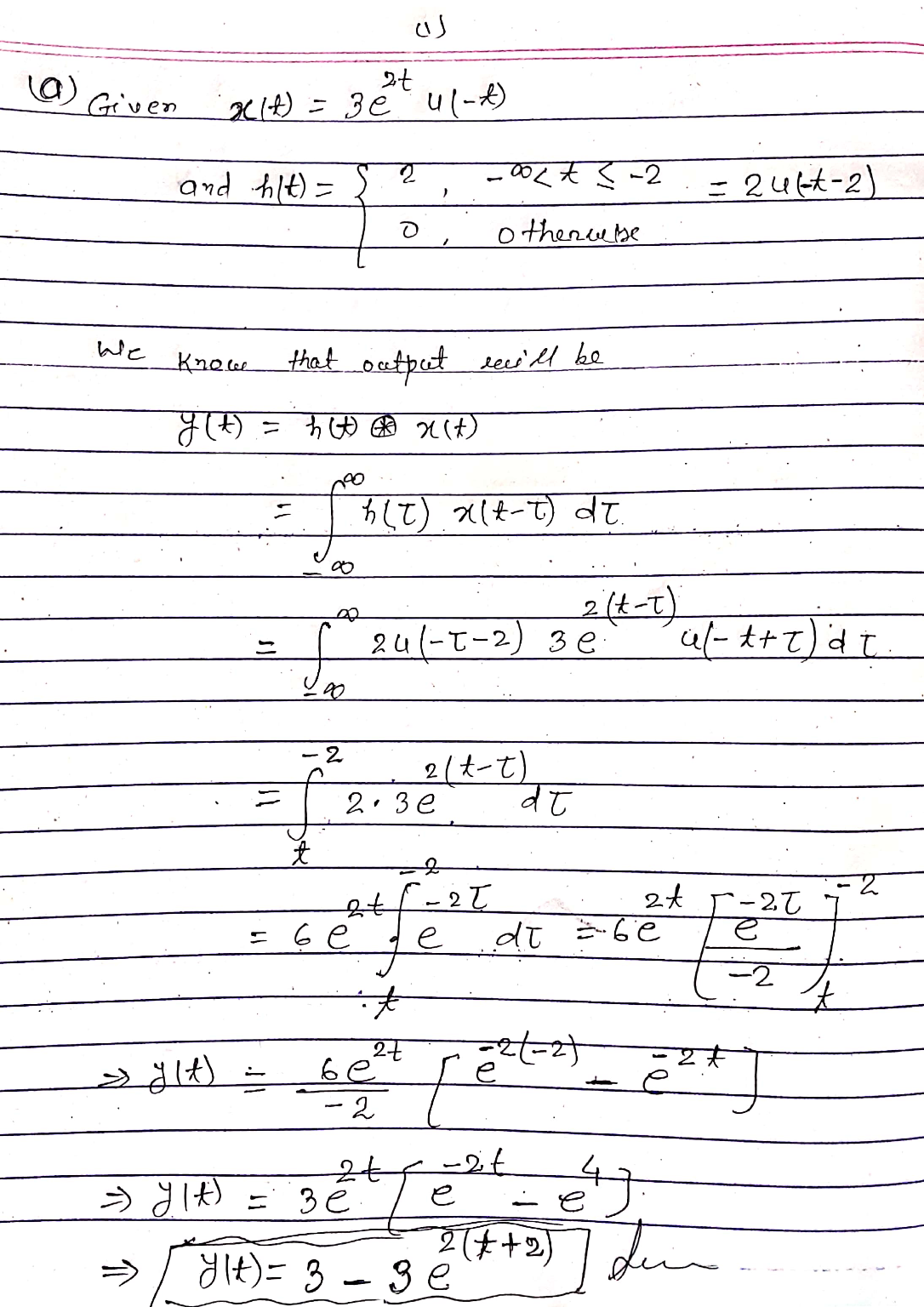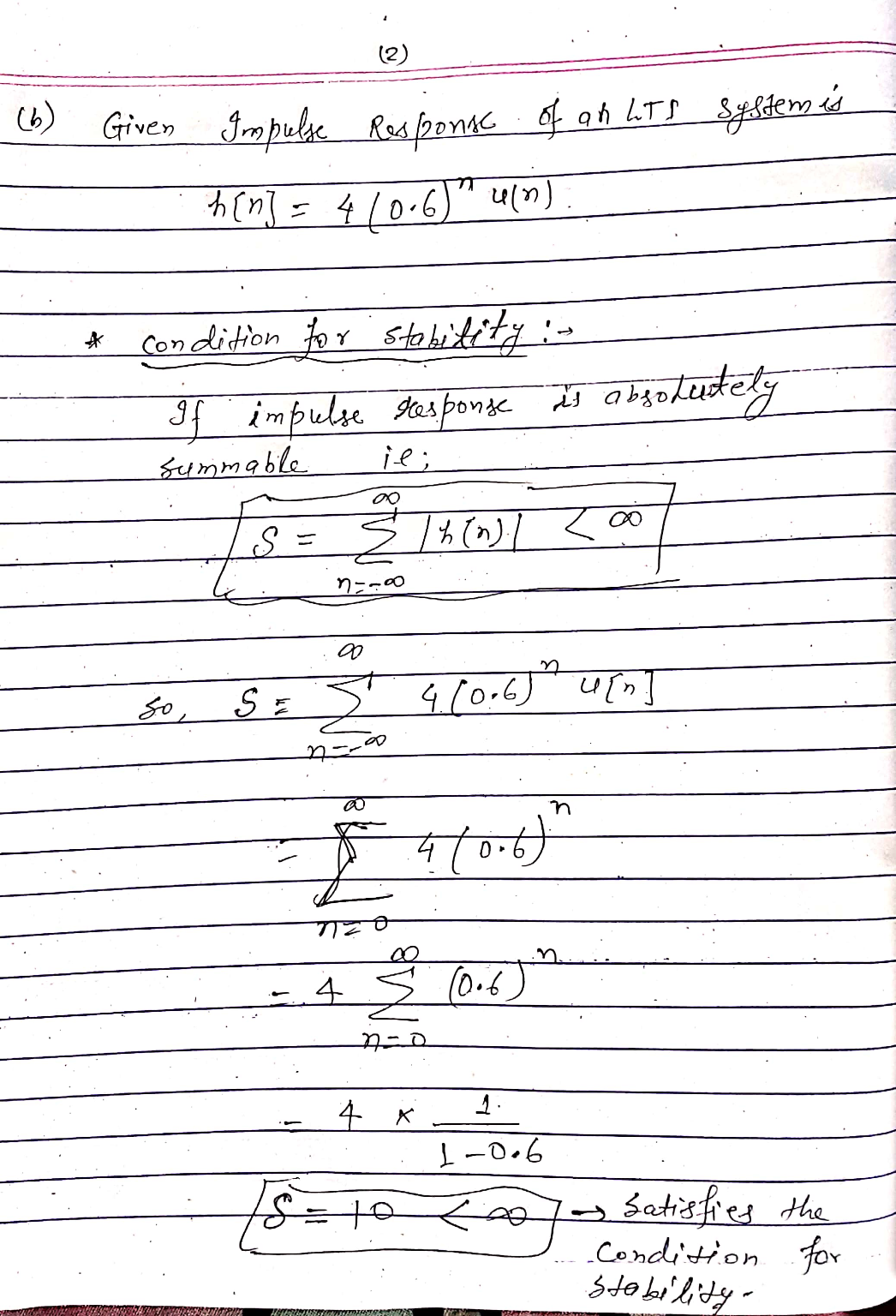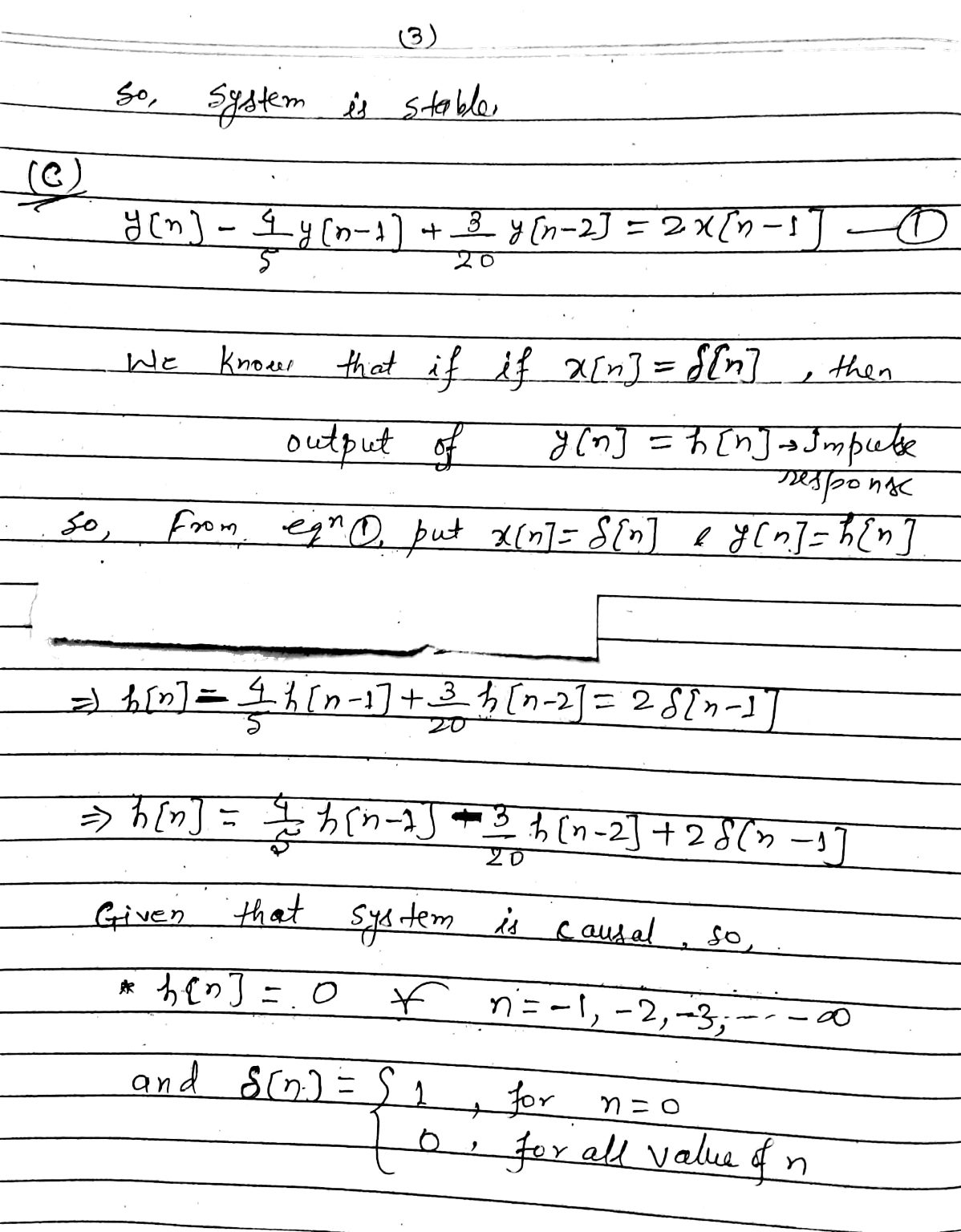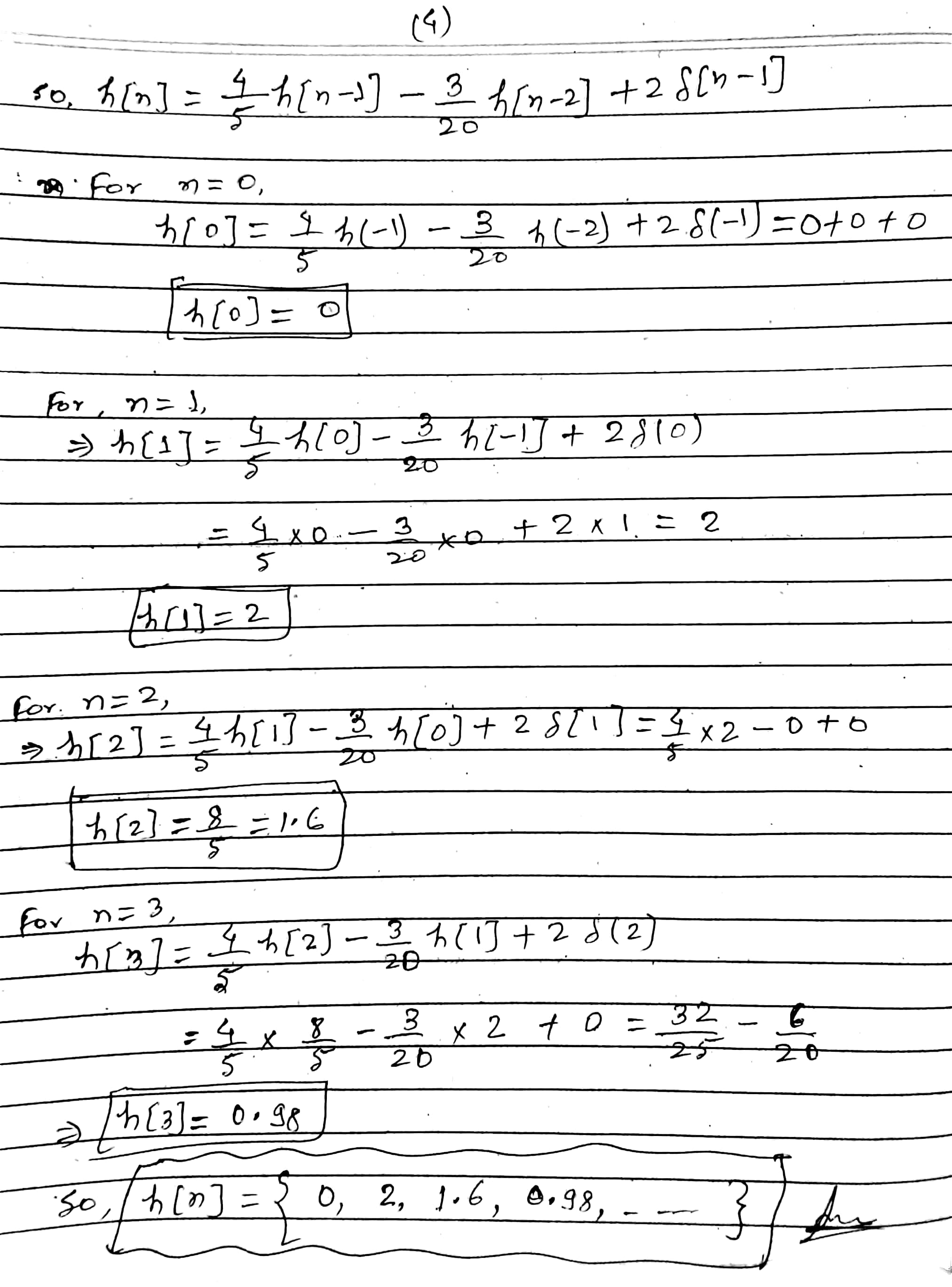#### Earn Coins

Coins can be redeemed for fabulous gifts.

Similar Homework Help Questions
• ### For a continuous time linear time-invariant system, the input-output relation is the following (x(t) the input, y(t) the...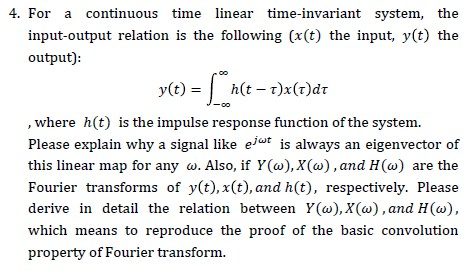For a continuous time linear time-invariant system, the input-output relation is the following (x(t) the input, y(t) the output): , where h(t) is the impulse response function of the system. Please explain why a signal like e/“* is always an eigenvector of this linear map for any w. Also, if ¥(w),X(w),and H(w) are the Fourier transforms of y(t),x(t),and h(t), respectively. Please derive in detail the relation between Y(w),X(w),and H(w), which means to reproduce the proof of the basic convolution property...

• ### A continuous-time LTI system has unit impulse response h(t). The Laplace transform of h(t), also called...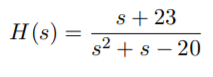A continuous-time LTI system has unit impulse response h(t). The Laplace transform of h(t), also called the “transfer function” of the LTI system, is . For each of the following cases, determine the region of convergence (ROC) for H(s) and the corresponding h(t), and determine whether the Fourier transform of h(t) exists. (a) The LTI system is causal but not stable. (b) The LTI system is stable but not causal. (c) The LTI system is neither stable nor causal 8...

• ### QUESTION 4 a. Determine the frequency spectrum of the signal x(t) b. What is the Nyquist...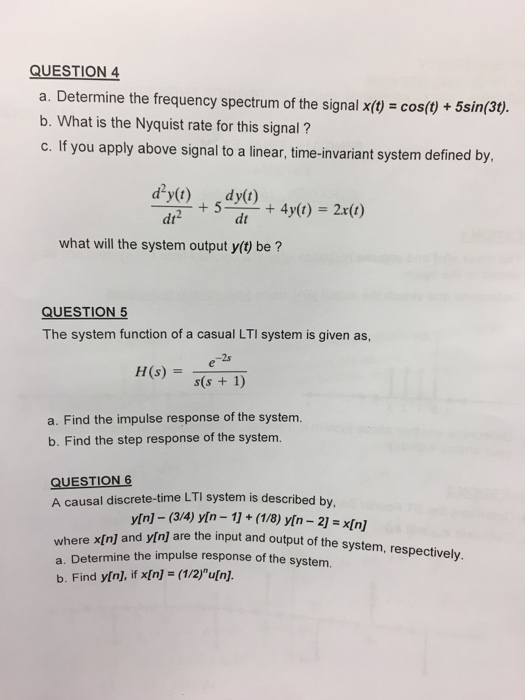QUESTION 4 a. Determine the frequency spectrum of the signal x(t) b. What is the Nyquist rate for this signal? cos(t) + 5sn3t. me-invariant system defined by, dt dt what will the system output y(t) be? QUESTION 5 The system function of a casual LTI system is given as, 2s a. Find the impulse response of the system. b. Find the step response of the system. A causal discrete-time LTI system is described by, y[n] - (3/4) yin-1+(1/8) yin-21 xn]...

• ### 2. Let y(t)(e')u(t) represent the output of a causal, linear and time-invariant continuous-time system with unit...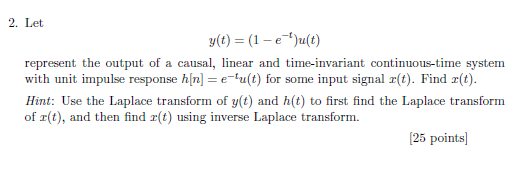2. Let y(t)(e')u(t) represent the output of a causal, linear and time-invariant continuous-time system with unit impulse response h[nu(t) for some input signal z(t). Find r(t) Hint: Use the Laplace transform of y(t) and h(t) to first find the Laplace transform of r(t), and then find r(t) using inverse Laplace transform. 25 points

• ### 2.6.1 Consider a causal continuous-time LTI system described by the differential equation u"(t) +...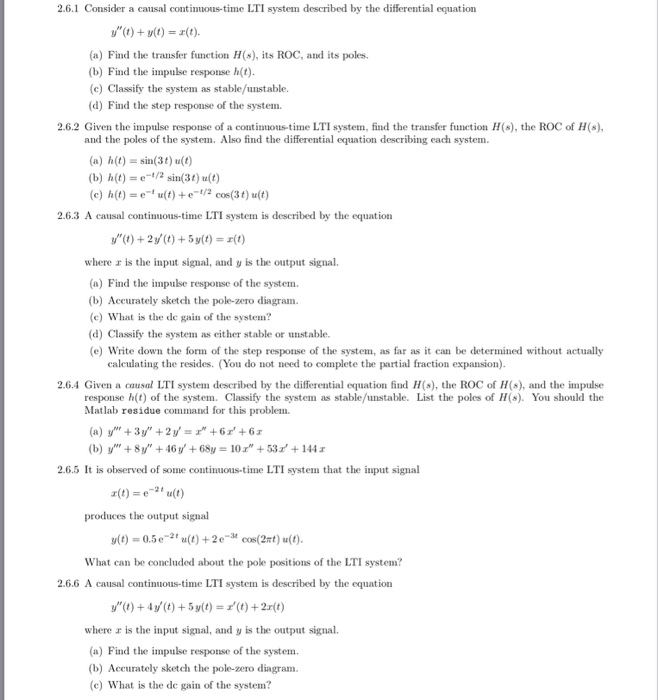2.6.1-2.6.62.6.1 Consider a causal contimuous-time LTI system described by the differential equation$$y^{\prime \prime}(t)+y(t)=x(t)$$(a) Find the transfer function $$H(s)$$, its $$R O C$$, and its poles.(b) Find the impulse response $$h(t)$$.(c) Classify the system as stable/unstable.(d) Find the step response of the system.2.6.2 Given the impulse response of a continuous-time LTI system, find the transfer function $$H(s),$$ the $$\mathrm{ROC}$$ of $$H(s)$$, and the poles of the system. Also find the differential equation describing each system.(a) $$h(t)=\sin (3 t) u(t)$$(b)...

• ### Question 1: (2 marks) Find the zero-input response yz(t) for a linear time-invariant (LTI) system described...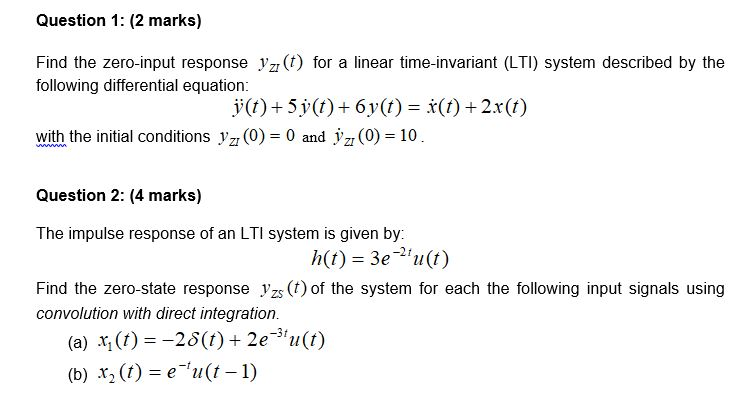Question 1: (2 marks) Find the zero-input response yz(t) for a linear time-invariant (LTI) system described by the following differential equation: j(t) + 5y(t) + 6y(t) = f(t) + 2x(t) with the initial conditions yz (0) = 0 and jz (0) = 10. Question 2: (4 marks) The impulse response of an LTI system is given by: h(t) = 3e?'u(t) Find the zero-state response yzs (t) of the system for each the following input signals using convolution with direct integration....

• ### If of the input signal X(t) and output signal yl to a continuous - time linear...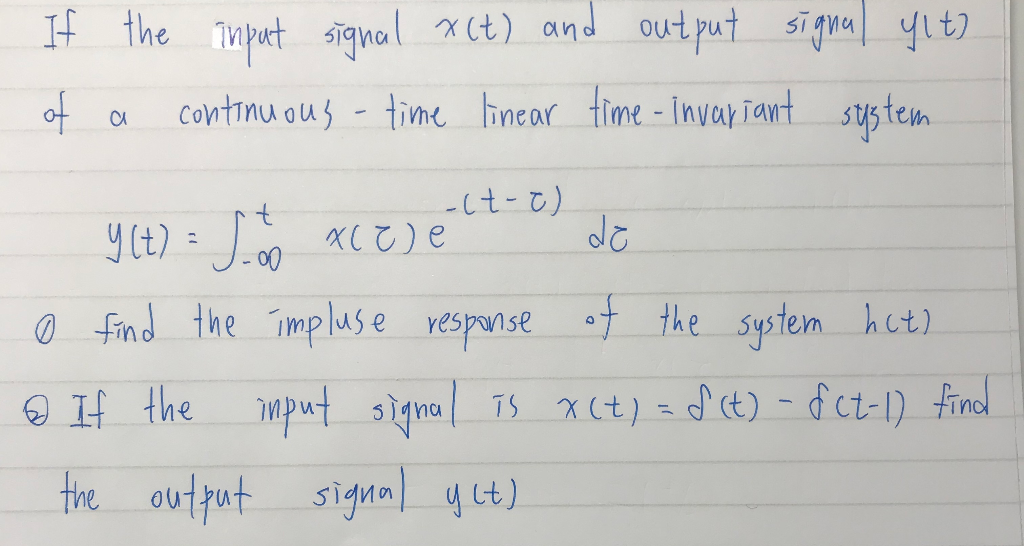If of the input signal X(t) and output signal yl to a continuous - time linear time - invariant system - Y(t) = 5 to xc ej elt- el de o find the impluse response of the system hat) o If the input signal is x (t) = f(t) - f (t-1) find the output signal y ct) o Find the impulse response of the system hit) @ If the input signal Is x (t) = f(t)-f(t-1) find the -...

• ### 2. (a) For each sample of a discrete time signal x[n] as input, a system S...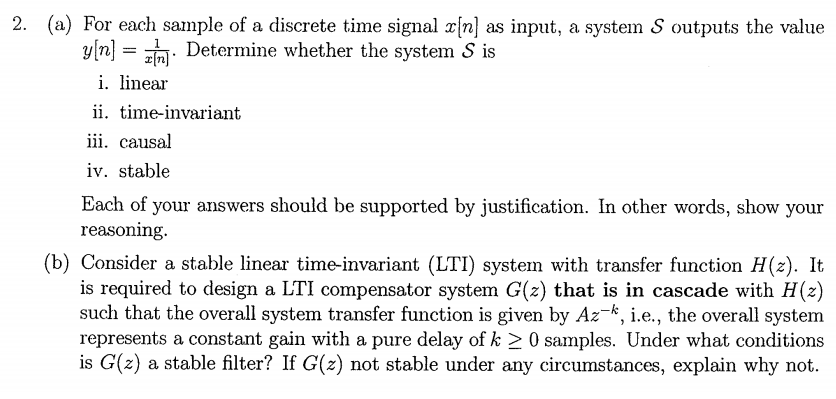2. (a) For each sample of a discrete time signal x[n] as input, a system S outputs the value y[n- . Determine whether the system S is i. linear ii. time-invariant 1ll. causal iv. stable Each of your answers should be supported by justification. In other words, show your reasoning (b) Consider a stable linear time-invariant (LTI) system with transfer function H(z). It is required to design a LTI compensator system G(z) that is in cascade with H(z) such that...

• ### 2. Short-answer questions on various topics (20 marks total) In each case, clearly explain the reasoning...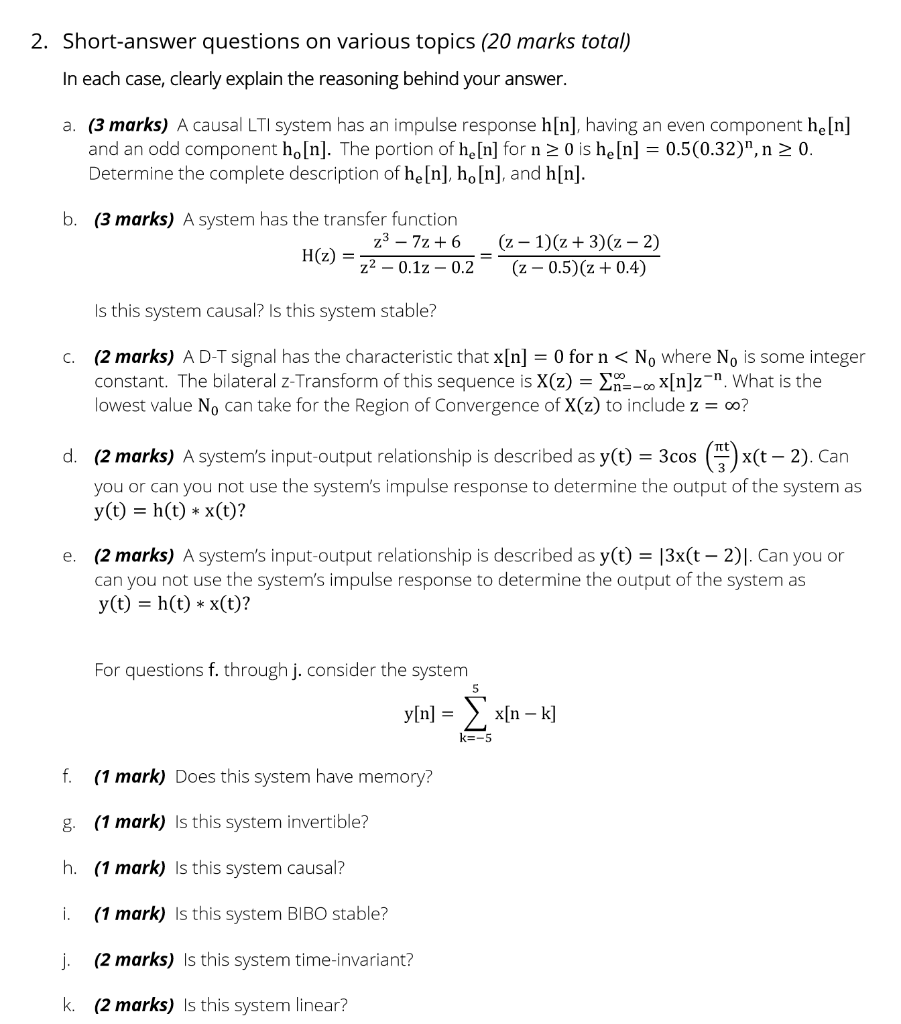2. Short-answer questions on various topics (20 marks total) In each case, clearly explain the reasoning behind your answer. a. (3 marks) A causal LTI system has an impulse response h[n], having an even component he[n] and an odd component ho[n]. The portion of he[n] for n > 0 is he[n] = 0.5(0.32)", n > 0. Determine the complete description of he[n], ho[n], and h[n]. b. (3 marks) A system has the transfer function z– 7z+6 H(2) = 22 -0.12...

• ### please answer both question 9 a. The wind sensor system used in the airplane is represented...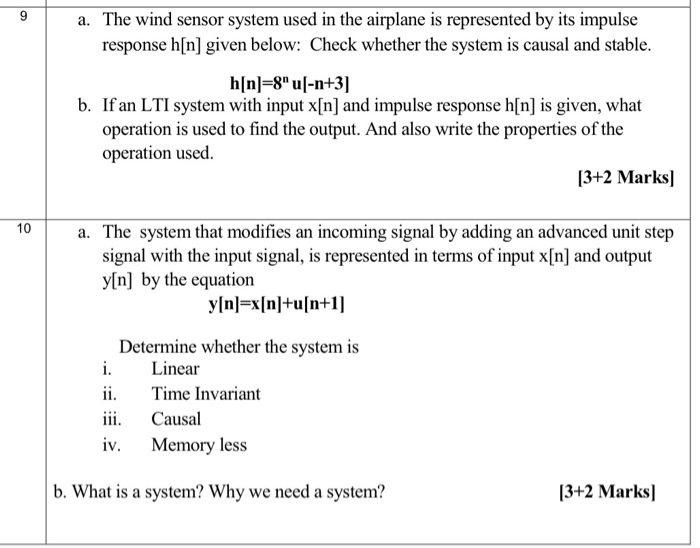please answer both question 9 a. The wind sensor system used in the airplane is represented by its impulse response h[n] given below: Check whether the system is causal and stable. h[n]=8" [-n+3] b. If an LTI system with input x[n] and impulse response h[n] is given, what operation is used to find the output. And also write the properties of the operation used. [3+2 Marks) 10 a. The system that modifies an incoming signal by adding an advanced unit...